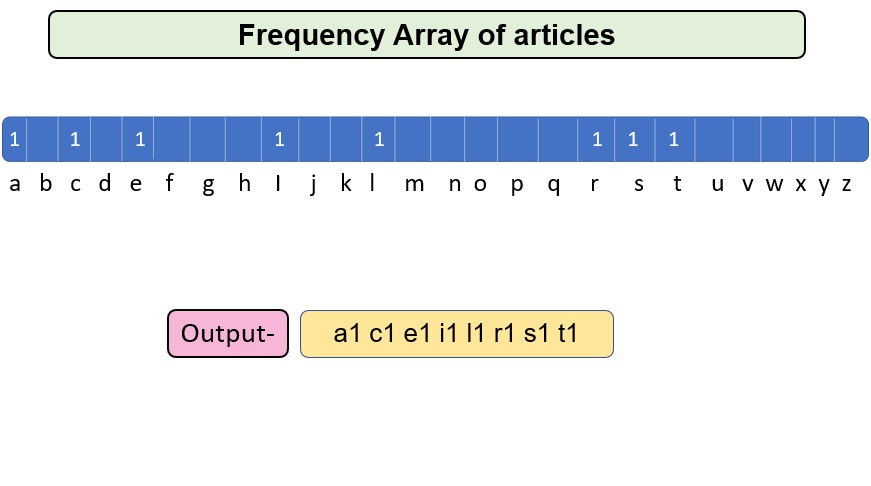# Calculate Frequency of characters in a String

Problem Statement: Given a string, calculate the frequency of characters in a string.

Examples:

```Example 1:
Input: takeuforward
Output: a2 d1 e1 f1 k1 o1 r2 t1 u1 w1
Explanation: Count of every character of string is printed.

Example 2:
Input: articles
Output: a1 c1 e1 i1 l1 r1 s1 t1
Explanation: Count of every character of string is printed.
```

### Solution

Disclaimer: Don’t jump directly to the solution, try it out yourself first.

Solution 1:

Approach: Sort the string and print the consecutive elements count.

For eg.

Str = articles

Sort the string first, the sorted string is aceilrst

Now, print the occurrences of every consecutive element – a1 c1 e1 i1 l1 r1 s1 t1

Code:

## C++ Code

``````#include <iostream>
#include <algorithm>
using namespace std;
void Printfrequency(string str)
{
sort(str.begin(), str.end());
char ch = str;
int count = 1;
for (int i = 1; i < str.length(); i++)
{
if (str[i] == ch)
count++;
else
{
cout << ch << count << " ";
count = 1;
ch = str[i];
}
}
cout << ch << count << " ";
}

int main()
{
string str = "takeuforward";
Printfrequency(str);
return 0;
}
``````

Output: a2 d1 e1 f1 k1 o1 r2 t1 u1 w1

Time Complexity: O(nlogn) since sorting function requires nlogn iterations.

Space Complexity: O(1)

## Java Coding

``````import java.util.*;
public class Main {
public static String SortString(String str) {
char c[] = str.toCharArray();
Arrays.sort(c);
return new String(c);
}

public static void Printfrequency(String str) {
str = SortString(str);
char ch = str.charAt(0);
int count = 1;
for (int i = 1; i < str.length(); i++) {
if (str.charAt(i) == ch)
count++;
else {
System.out.print(ch);
System.out.print(count + " ");
ch = str.charAt(i);
count = 1;
}
}
System.out.print(ch);
System.out.print(count + " ");
}

public static void main(String args[]) {
String str = "takeuforward";
Printfrequency(str);
}
}``````

Output: a2 d1 e1 f1 k1 o1 r2 t1 u1 w1

Time Complexity: O(nlogn) since sorting function requires nlogn iterations.

Space Complexity: O(1)

Solution 2:

Approach: Just count the frequency of every element in Str1 and iterate through the frequency array and print the count of every letter in the string.

For Eg –

Str = articles

The frequency array of every element of Str is  :What is frequency array?

It’s simple, since we know that the string contains only lowercase english alphabets, there are only 26 such characters possible. Now, we need an array of size 26 to store frequency of characters in the original string. This array is the frequency array.

How will this frequency array work?

Since we have only 26 possible lowercase english alphabets. The indexes of the array will represent all such characters and value at any index will represent the count or frequency of that character in the original array.

For example, index 0 will represent character ‘a’, index 1 will represent the character ‘b’, so on and so forth.

Code:

## C++ Code

``````#include <iostream>
using namespace std;

void Printfrequency(string str)
{
int freq = {0};
for (int i = 0; i < str.length(); i++)
{
freq[str[i] - 'a']++;
}
for (int i = 0; i < 26; i++)
{
if (freq[i] != 0)
{
cout << (char)(i + 'a') << freq[i] << " ";
}
}
}

int main()
{
string str = "takeuforward";
Printfrequency(str);
return 0;
}``````

Output: a2 d1 e1 f1 k1 o1 r2 t1 u1 w1

Time Complexity: O(n) where n is the length of string

Space Complexity: O(1)

## Java Code

``````import java.util.*;
public class Main
{
public static void Printfrequency(String str)
{
int[] freq = new int;
for (int i = 0; i < str.length(); i++)
{
freq[str.charAt(i) - 'a']++;
}
for (int i = 0; i < 26; i++)
{
if (freq[i] != 0)
{
System.out.print((char)(i + 'a'));
System.out.print(freq[i] + " ");
}
}
}

public static void main(String args[])
{
String str = "takeuforward";
Printfrequency(str);
}
}``````

Output: a2 d1 e1 f1 k1 o1 r2 t1 u1 w1

Time Complexity: O(n) where n is the length of string

Space Complexity: O(1)

Special thanks to Gurmeet Singh for contributing to this article on takeUforward. If you also wish to share your knowledge with the takeUforward fam, please check out this article